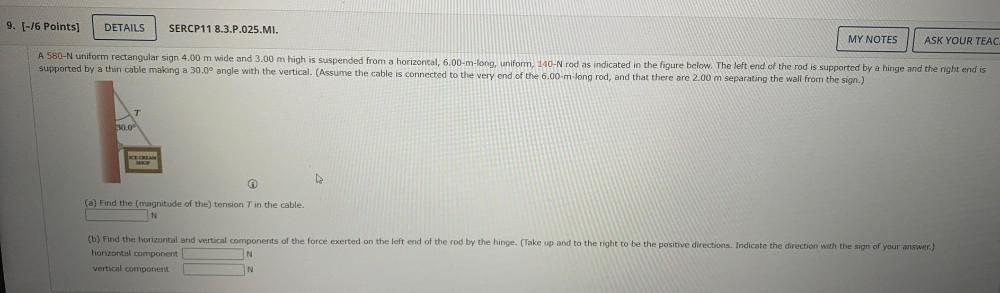Question:

# A 580-N uniform rectangular sign 4.00 m wide and 3.00 m high is suspended from a horizontal, 6.00-m-long, uniform, 140-N rod as indicated in the figure below. The left end of the rod is supported by aA 580-N uniform rectangular sign 4.00 m wide and 3.00 m high is suspended from a horizontal, 6.00-m-long, uniform, 140-N rod as indicated in the figure below. The left end of the rod is supported by a hinge and the right end is supported by a thin cable making a 30.0° angle with the vertical. (Assume the cable is connected to the very end of the 6.00-m-long rod, and that there are 2.00 m separating the wall from the sign.) (a) Find the (magnitude of the) tension T in the cable. (b) Find the horizontal and vertical components of the force exerted on the left end of the rod by the hinge. (Take up and to the right to be the positive directions. Indicate the direction with the sign of your answer.)